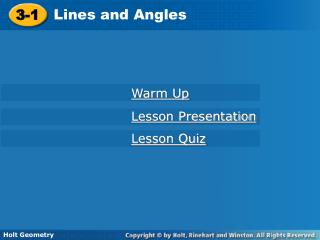DownloadDownload Presentation3-1

# 3-1

Download Presentation## 3-1

- - - - - - - - - - - - - - - - - - - - - - - - - - - E N D - - - - - - - - - - - - - - - - - - - - - - - - - - -
##### Presentation Transcript

1. 3-1 Lines and Angles Holt Geometry Warm Up Lesson Presentation Lesson Quiz

2. Warm Up Identify each of the following. 1.points that lie in the same plane 2. two angles whose sum is 180° 3. the intersection of two distinct intersecting lines 4. a pair of adjacent angles whose non-common sides are opposite rays

3. Objectives Identify parallel, perpendicular, and skew lines. Identify the angles formed by two lines and a transversal.

4. Vocabulary parallel lines perpendicular lines skew lines parallel planes transversal corresponding angles alternate interior angles alternate exterior angles same-side interior angles

5. Helpful Hint Segments or rays are parallel, perpendicular, or skew if the lines that contain them are parallel, perpendicular, or skew.

6. Example 1: Identifying Types of Lines and Planes Identify each of the following. A. a pair of parallel segments B. a pair of skew segments C. a pair of perpendicular segments D. a pair of parallel planes

7. Check It Out! Example 1 Identify each of the following. a. a pair of parallel segments b. a pair of skew segments c. a pair of perpendicular segments d. a pair of parallel planes

8. Example 2: Classifying Pairs of Angles Give an example of each angle pair. A. corresponding angles B. alternate interior angles C. alternate exterior angles D. same-side interior angles

9. Check It Out! Example 2 Give an example of each angle pair. A. corresponding angles B. alternate interior angles C. alternate exterior angles D. same-side interior angles

10. Helpful Hint To determine which line is the transversal for a given angle pair, locate the line that connects the vertices.

11. Example 3: Identifying Angle Pairs and Transversals Identify the transversal and classify each angle pair. A. 1 and 3 B. 2 and 6 C. 4 and 6

12. Check It Out! Example 3 Identify the transversal and classify the angle pair 2 and 5 in the diagram.

13. Lesson Quiz: Part I Identify each of the following. 1. a pair of parallel segments 2. a pair of skew segments 3. a pair of perpendicular segments 4. a pair of parallel planes

14. Lesson Quiz: Part II Identify each of the following. 5. one pairalternate interior angles 6. One pair corresponding angles 7. one pairalternate exterior angles 8. one pairsame-side interior angles